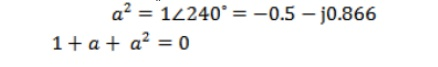Home | | Power System Analysis | Introduction to Symmetrical Components

# Introduction to Symmetrical Components

Symmetrical components of a 3 phase system

INTRODUCTION TO SYMMETRICAL COMPONENTS

## Symmetrical components of a 3 phase system

In a 3 phase system, the unbalanced vectors (either currents or voltage) can be resolved into three balanced system of vectors.

They are Positive sequence components Negative sequence components

Zero sequence components

Unsymmetrical fault analysis can be done by using symmetrical components.

## Positive sequence components

It consists of three components of equal magnitude, displaced each other by 120˚ in phase and having the phase sequence abc .## Negative sequence components

It consists of three components of equal magnitude, displaced each other by 120˚ in phase and having the phase sequence acb .## Zero sequence components

It consists of three phasors equal in magnitude and with zero phase displacement from each other.## Sequence operator

In unbalanced problem, to find the relationship between phase voltages and phase currents, we use sequence operator ‘a’.

= 1120˚ == - 0.5+j0.86## Unbalanced currents from symmetrical currents

Let, Ia, Ib, Ic be the unbalanced phase currents

Let, Ia0, Ia1, Ia2 be the symmetrical components of phase a## Determination of symmetrical currents from unbalanced currents.

Let, Ia, Ib, Ic be the unbalanced phase currents

Let, Ia0, Ia1, Ia2 be the symmetrical components of phase aStudy Material, Lecturing Notes, Assignment, Reference, Wiki description explanation, brief detail
Power System Analysis : Symmetrical Components And Unbalanced Fault Analysis : Introduction to Symmetrical Components |